## A vehicle with a mass of 1250.0 kg accelerates from 0 to 50 km/h in 10.0 s. What is the net force on the vehicle?

Question

A vehicle with a mass of 1250.0 kg accelerates from 0 to 50 km/h in 10.0 s. What is the net force on the vehicle?

in progress 0
2 months 2021-07-23T07:57:09+00:00 1 Answers 4 views 0

1,736.1N

Explanation:

The information we have is:

mass: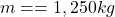initial velocity: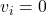final velocity: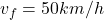we need to converto to m/s: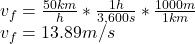time: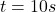.

First we need to calculate the acceleration: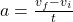substituting the values: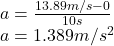and now we use Newton’s second law to calculate the net force: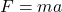substituting the mass and acceleration: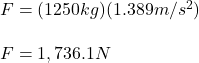the net force on the vehicle is in Newtons is: 1,736.1N